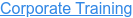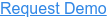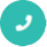#### Digital Marketing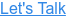# Top Python Interview Questions

by Venkatesan M, on Sep 20, 2019 10:51:35 AMAre you trying for a Python job? Here are the top frequently asked interview questions and answers to step-on the python interview. Dive into these Python interview questions and answers and see just how well-versed you are in this Python language.

## Q1. What is the maximum possible length of an identifier?

a) 31 characters

b) 63 characters

c) 79 characters

d) none of the mentioned

Explanation: Identifiers can be of any length.

## Q2. Why are local variable names beginning with an underscore discouraged?

a) they are used to indicate a private variable of a class

b) they confuse the interpreter

c) they are used to indicate global variables

d) they slow down execution

Explanation: As Python has no concept of private variables, leading underscores are used to indicate variables that must not be accessed from outside the class.

## Q3. What is the output of print 0.1 + 0.2 == 0.3?

a) True

b) False

c) Machine dependent

d) Error

Explanation: Neither of 0.1, 0.2 and 0.3 can be represented accurately in binary. The round off errors from 0.1 and 0.2 accumulate and hence there is a difference of 5.5511e-17 between (0.1 + 0.2) and 0.3

## Q4. What is the result of round(0.5) – round(-0.5)?

a) 1.0

b) 2.0

c) 0.0

d) None of the mentioned

Explanation: Python rounds off numbers away from 0 when the number to be rounded off is exactly halfway through. round(0.5) is 1 and round(-0.5) is -1.

## Q5. What is the output of the following expression if x=456?

print("%-06d"%x)

a) 000456

b) 456000

c) 456

d) error

Explanation: The expression shown above results in the output 456.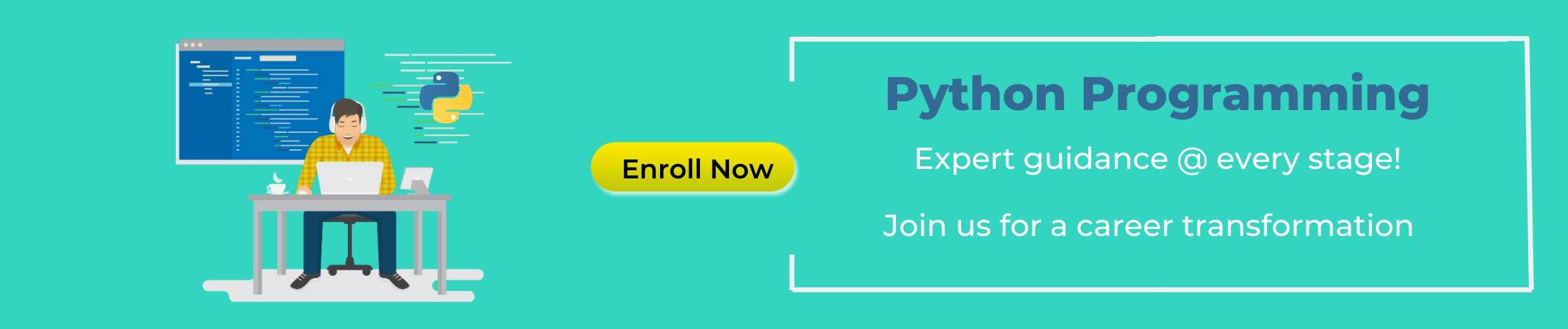## Q6. What is the output of the following?

i = 0

while i < 3:

print(i)

i += 1

else:

print(0)

a) 0 1 2 3 0

b) 0 1 2 0

c) 0 1 2

c) error

Explanation: The else part is executed when the condition in the while statement is false

## Q7. What is the output of the following?

x = "abcdef"

i = "a"

while i in x[:-1]:

print(i, end = " ")

a) a a a a a

b) a a a a a a

c) a a a a a a …

d) a

Explanation: String x is not being altered and i is in x[:-1].

## Q8. What is the output of the following?

print('ab12'.isalnum())

a) True

b) False

c) None

d) Error

Explanation: The string has only letters and digits.## Q9. Suppose listExample is [3, 4, 5, 20, 5, 25, 1, 3], what is list1 after list example.pop()?

a) [3, 4, 5, 20, 5, 25, 1].

b) [1, 3, 3, 4, 5, 5, 20, 25].

c) [3, 5, 20, 5, 25, 1, 3].

d) [1, 3, 4, 5, 20, 5, 25].

Explanation: pop() by default will remove the last element.

## Q10. What is the output of the code shown below?

l1=[1,2,3]

l2=[4,5,6]

[x*y for x in l1 for y in l2]

a) [4, 8, 12, 5, 10, 15, 6, 12, 18]

b) [4, 10, 18]

c) [4, 5, 6, 8, 10, 12, 12, 15, 18]

d) [18, 12, 6, 15, 10, 5, 12, 8, 4]

Explanation: The code is shown above returns x*y, where x belongs to the list l1 and y belongs to the list l2. Therefore, the output is: [4, 5, 6, 8, 10, 12, 12, 15, 18].

## Q11. Which of the following statements create a dictionary?

a) d = {}

b) d = {“john”:40, “peter”:45}

c) d = {40:”john”, 45:”peter”}

d) All of the mentioned

Explanation: Dictionaries are created by specifying keys and values.

## Q12. What is the output of the below program?

lamb = lambda x: x ** 3

print(lamb(5))

a) 15

b) 555

c) 125

d) None of the mentioned

## Q13. What is the value returned by Math.floor(3.4)?

a) 3

b) 4

c) 4.0

d) 3.0

Explanation: The floor function returns the biggest number that is smaller than or equal to the number itself.

## Q14. What is the output of the code shown below?

l=[2, 3, [4, 5]]

l2=l.copy()

l2=88

l

l2

a) [88, 2, 3, [4, 5]]

[88, 2, 3, [4, 5]]

b) [2, 3, [4, 5]]

[88, 2, 3, [4, 5]]

c) [88, 2, 3, [4, 5]]

[2, 3, [4, 5]]

d) [2, 3, [4, 5]]

[2, 3, [4, 5]]

Explanation: The code shown above depicts a deep copy. In a deep copy, the base address of the objects is not copied. Hence the modification done on one list does not affect the other list.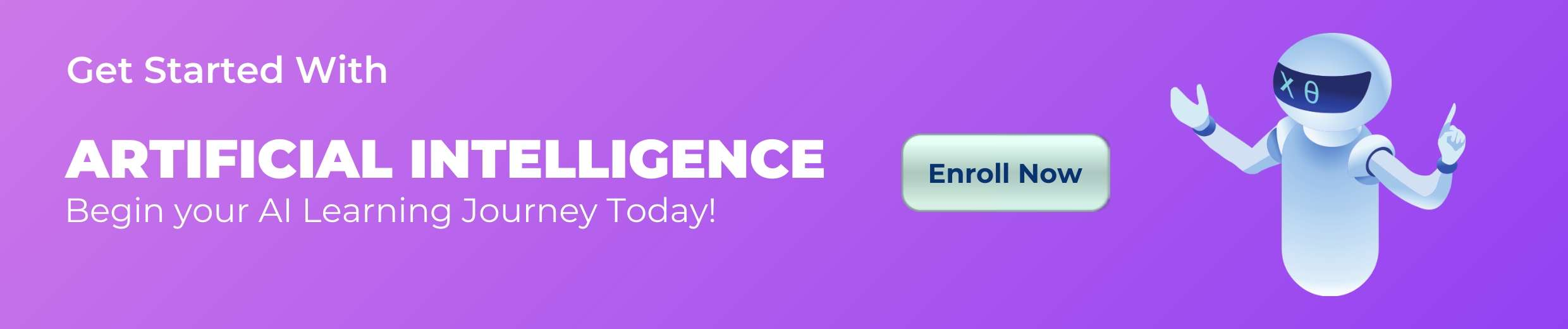Related Interview Questions...